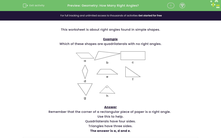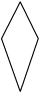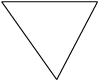# Geometry: How Many Right Angles?

In this worksheet, students state which of the quadrilaterals and triangles shown have right angles (angles of 90 degrees).Key stage:  KS 2

Curriculum topic:   Geometry: Properties of Shapes

Curriculum subtopic:   Identify Acute/Obtuse Angles

Popular topics:   Angles worksheets, Geometry worksheets

Difficulty level:#### Worksheet Overview

This activity is about right angles found in simple shapes.

Example

Which of these shapes are quadrilaterals with no right angles.abcdefgh

Remember that the corner of a rectangular piece of paper is a right angle.

Triangles have three sides.

So, the quadrilaterals above are shapes a, c, d and e.

The only one of those four quadrilaterals that has right angles is c, which is a rectangle.

This means that a, d and e are the quadrilaterals above that have no right angles.Let's have a go at some questions now.

### What is EdPlace?

We're your National Curriculum aligned online education content provider helping each child succeed in English, maths and science from year 1 to GCSE. With an EdPlace account you’ll be able to track and measure progress, helping each child achieve their best. We build confidence and attainment by personalising each child’s learning at a level that suits them.

Get started